The Learning Point‎ > ‎Mathematics‎ > ‎

### Triangles and Congruence

Theorems:
1.1 Definition:Two triangles are congruent if their corresponding sides are equal in length and their corresponding angles are equal in size.

1.2 There are five basic condition that can be used to compare the congruency of triangles.They are as following:

1.SAS(Side-Angle-Side):If two pairs of sides of two triangles are equal in length, and the included angles are equal in measurement, then the triangles are congruent.It is illustrated by the folowing figure.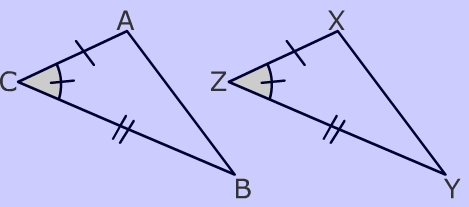2.SSS(Side-Side-Side):If three pairs of sides of two triangles are equal in length, then the triangles are congruent.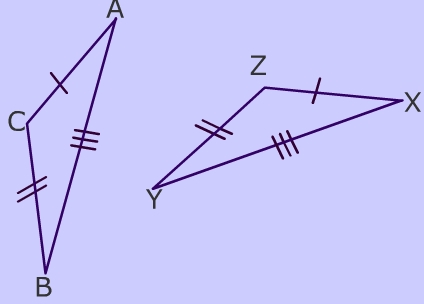3.ASA(Angle-Side-Angle):If two pairs of angles of two triangles are equal in measurement, and the included sides are equal in length, then the triangles are congruent.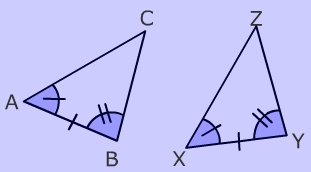4.AAS(Angle-Angle-Side):If two pairs of angles of two triangles are equal in measurement, and a pair of corresponding non-included sides are equal in length, then the triangles are congruent.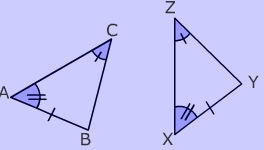5.RHS(Right-angle-Hypotenuse-Side):If two right-angled triangles have their hypotenuses equal in length, and a pair of shorter sides are equal in length, then the triangles are congruen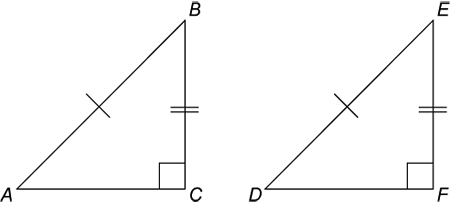1.3 Problems: (Hints/Solutions at the end)
Q1.In the figure below, PX and QY are perpendicular to PQ and PX = QY. Show that AX = AY.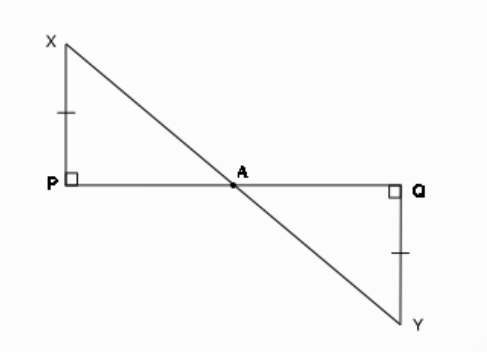Q2.In figure below, ∆ABC is a right triangle in which ∠B = 90° and D is the midpoint of AC.Prove that BD = ½ AC.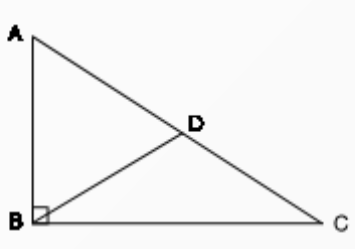Q3.In following figure,  ∠B =  ∠C and AB = AC. Prove that  ∆ABE  ≅ ∆ACD. Hence show that CD = BE.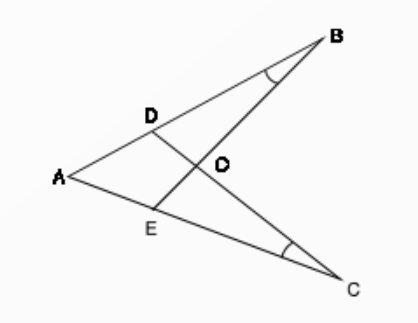Q4.ABC is an isosceles triangle in which AB = AC. If BD ⊥ AC and CE ⊥ AB, prove that BD = CE.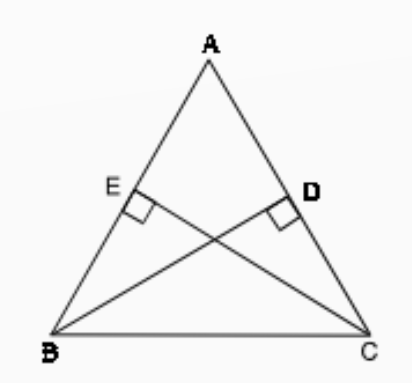Q5.In the following Figure, ∆ABC and ∆CDE are such that BC = CE and AB = DE. If ∠B = 60°, ∠ACE = 30° and ∠D = 90°, then prove that the two triangles are congruent.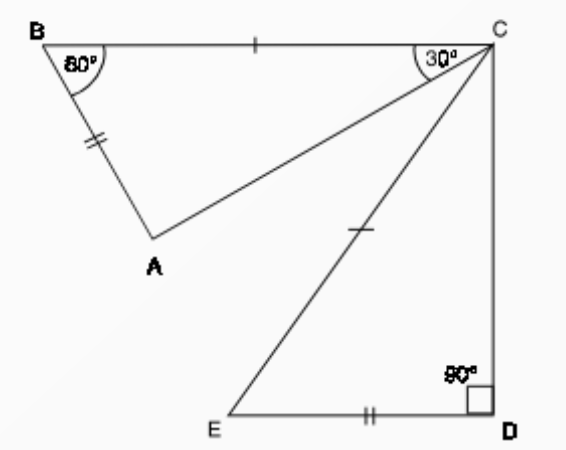Q6.In the following Fig., AB is the longest side and DC is the shortest
side of a quadrilateral ABCD. Prove that  ∠C > ∠A and ∠D > ∠B.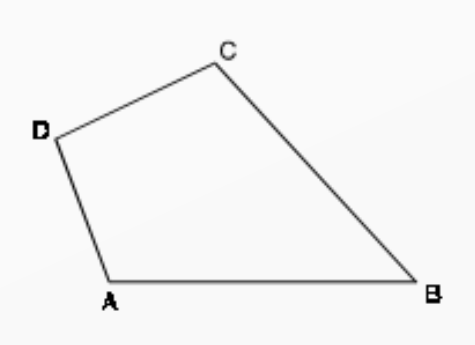Hints and Solutions:
Problem 1. The two triangles are congruent using AAS congruence. Hence the length of the hypotenuse will be equal for both of them.
Problem 2. Drop a perpendicular from D to BC, which meets BC at a point X. Triangles BXD and CXD are congruent by ASA. BD=CD=AC/2
Problem 3. Prove both triangles ABE and ACD congruent by ASA.
Problem 4. Triangles BEC and BDC are congruent by AAS (right angles, equal base angles, common side BC) => from this you may show that BD = EC
Problem 5. Both triangles are congruent by RHS